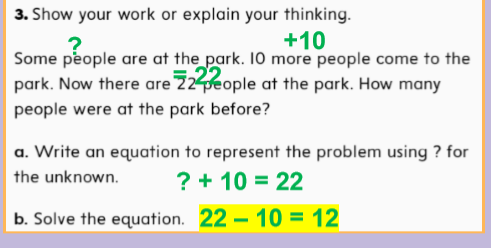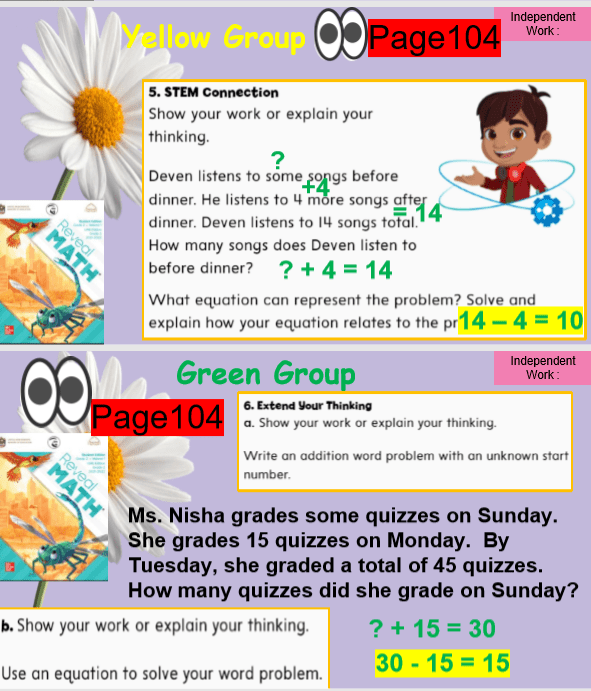# حل درس represent and solve problems الرياضيات المتكاملة الصف الثاني

حل درس represent and solve problems الرياضيات المتكاملة الصف الثاني

PAGE 101
Represent and Solve Problems
Ms. Nicole

Success Criteria:
I can solve problems using equations!

part-part whole:
To add the same number repeatedly.
3 + 3 + 3 = 9

unknown:
To add the same number repeatedly.
3 + 3 + 3 = 9

How many crayons were there at first?
How many are there now?

Ms. Edwards has some crayons in a box. She put some crayons in the box.
Now there are more crayons in the box.

There were 43 cows standing on the hill. Some more cows joined them. Then there were 48 cows. How many cows joined?
43 +5 = 48تصفح أيضا: